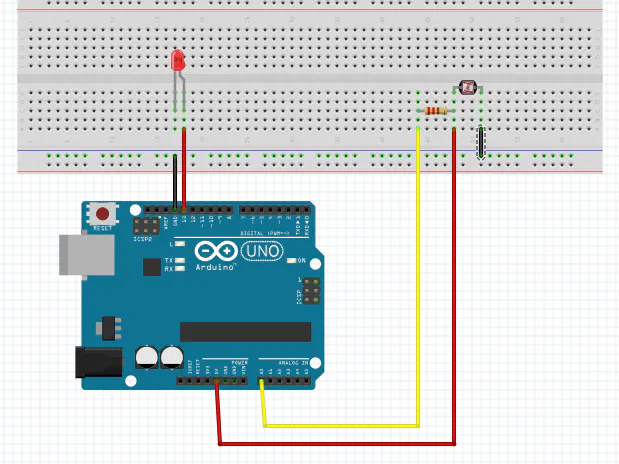Project tutorialUsing Sensors with Arduino © GPL3+

This is a tutorial on how to use sensors with Arduino.

• 182 views
• 2 respects

Apps and online services

Sensors are widely used in almost every field. Sensors give another dimension to your project and can be used in infinite applications. So, I decided to make this tutorial to help those new to sensors. I hope this will help you understand better. Let begin!

Firstly, there are different type of sensors which can record different type of data. All sensors work differently e.g. light sensors, ultrasonic sensor, gas sensor, humidity sensors etc.

Although all sensors work differently but when it come to interfacing these sensors with a micro controller (Arduino Uno in our case) the process is pretty much the same.

So let us understand how a sensor works?

Sensor record the physical data by changing the voltage at their output pin in response to different physical condition. Lets understand this with an example.

Consider a light sensor (LDR). When it's in a dark environment the output voltage is low but when we place it in a brighter environment it's output voltage increases.

Now, this change in voltage is noted by micro controller and it can programmed to respond accordingly.

In this tutorial we will be interfacing a LDR with Arduino Uno.

Connections

Remember there are two types of signals:

1. Digital Signals: These signals have only two values i.e. 1 or 0 (on or off).

2. Analog Signals: These signals have values in a range. In the case of Arduino it scales the value in the range from 0 to 255.

So, we will connect our LDR with A0 pin of the Arduino Uno.

For better understanding we will control an LED on the basis of the sensor data.

Connect the LED to D13 (digital pin 13) of Arduino UNO.

Note that here we are connecting our LED to pin 13 which has an inbuilt resistor. So we don't need to connect an external resistor but if we use any other pin then you will have to connect an external resistor to protect you LED.

So sensors simply records the data and sends it to then Arduino Uno which generates signals to control the LED. Now, let us see the code.

• CODE
• -------------------------------------------------------------------------------------

First, we initialize an integer variable to store the data sent by the sensor.

int sensor1Value = 0;

void setup()

{

// Declare the ledPin13 as an OUTPUT:

pinMode(13, OUTPUT);

}

void loop() {

// nowwesetupaconditionandcheck the value of sensor

if(sensor1Value <200)

{

//If the value is less than 200 then turn the LEDs on

digitalWrite(13, HIGH);

delay(500);

}

else

//If the value is greater than or equal to 200 then turn LEDs off.

{

digitalWrite(13, LOW);

delay(500);

}

}

--------------------------------------------------------------------------------------

That's it!

I hope this tutorial will be helpful to you and it made sensors clear.

Just plug in your Arduino, make those connections and you are good to go!

Code

Using LDR with ArduinoC/C++
You will have to upload this code on to Arduino Uno.
```int sensor1Value = 0;
void setup()
{
// declare the ledPins as an OUTPUT:
pinMode(13, OUTPUT);

}

void loop() {
// read the value from the sensor:
{
if(sensor1Value <200)     // check the value of sensor
{                          //if the value is less than 200 then turn the leds on
digitalWrite(13, HIGH);
delay(500);
}
else                      // if the value is greater than or equal to 200 then turn leds off
{
digitalWrite(13, LOW);
delay(500);
}
}
```

Schematics

This is how the connection is to be made.Published on

November 30, 2018

Members who respect this project

See similar projects
you might like

Test DHT22 Sensors with Arduino and MATLAB

Project tutorial by dancili

• 4,468 views
• 20 respects

by Robiuri

• 4,698 views
• 9 respects

• 3,403 views
• 6 respects

Standalone Arduino Applied in Projects

Project tutorial by PCBWay and Silícios Lab

• 5,605 views
• 40 respects

How to Use Modbus with Arduino

Project tutorial by hwhardsoft

• 5,374 views